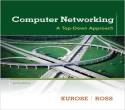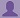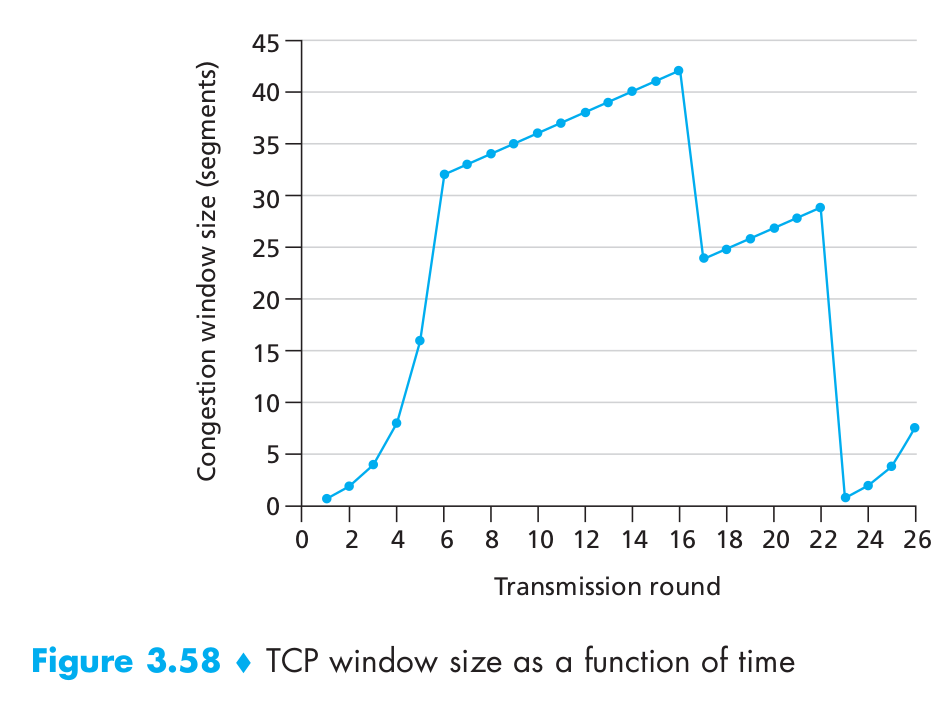SHARE
HELP

To start with, Sr2Jr’s first step is to reduce the expenses related to education. To achieve this goal Sr2Jr organized the textbook’s question and answers. Sr2Jr is community based and need your support to fill the question and answers. The question and answers posted will be available free of cost to all.# Computer Networking : A Top-down Approach

Authors:
James F. Kurose, Keith W. Ross
Exercise:
Problems
Chapter:
Transport Layer
Edition:
6
ISBN:
9780132856201
Question:
40
 Previous Next

###Question

Consider Figure 3.58. Assuming TCP Reno is the protocol experiencing the behavior shown above, answer the following questions. In all cases, you should provide a short discussion justifying your answer.

a. Identify the intervals of time when TCP slow start is operating.

b. Identify the intervals of time when TCP congestion avoidance is operating.

c. After the 16th transmission round, is segment loss detected by a triple duplicate ACK or by a timeout?

d. After the 22nd transmission round, is segment loss detected by a triple duplicate ACK or by a timeout?

e. What is the initial value of ssthresh at the first transmission round?

f. What is the value of ssthresh at the 18th transmission round?

g. What is the value of ssthresh at the 24th transmission round?

h. During what transmission round is the 70th segment sent?

i. Assuming a packet loss is detected after the 26th round by the receipt of a triple duplicate ACK, what will be the values of the congestion window size and of ssthresh?

j. Suppose TCP Tahoe is used (instead of TCP Reno), and assume that triple duplicate ACKs are received at the 16th round. What are the ssthresh and the congestion window size at the 19th round?

k. Again suppose TCP Tahoe is used, and there is a timeout event at 22nd round. How many packets have been sent out from 17th round till 22nd round, inclusive?###Answer

a) If TCP slow start is operating, then the intervals of time 1 to 6  and 23 to 26.

b) If TCP congestion avoidance is operating, then the intervals of time 6 to 23.

c) After the 16th transmission round, then the segment loss detected by a triple duplicate ACK.

d) After the 22nd transmission round, then the segment loss detected by timeout.

e) The initial value of ssthresh at the first transmission round 32.

f) The value of ssthresh at the 18th transmission round 21.

g) The value of ssthresh at the 24th transmission round 13.

h) The transmission round is the 70th segment sent is 7.

i) If a packet loss is detected after the 26th round by the receipt of a triple duplicate ACK, then the value is 4.

j) Suppose TCP Tahoe is used (instead of TCP Reno), and assume that triple duplicate ACKs are received at the 16th round. Then the ssthresh and the congestion window size at the 19th round is 1 and transmission round is 21.

k) Again suppose TCP Tahoe is used, and there is a timeout event at 22nd round, then the packets have been sent out from 17th round till 22nd round(inclusive) is 52

### Discussions

Post the discussion to improve the above solution.